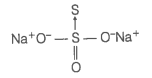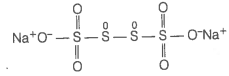23. Calculate the oxidation number of each sulphur atom in the following compounds.

(a) ${\mathrm{Na}}_{2}{\mathrm{S}}_{2}{\mathrm{O}}_{3}$

(b) ${\mathrm{Na}}_{2}{\mathrm{S}}_{4}{\mathrm{O}}_{6}$

(c) ${\mathrm{Na}}_{2}{\mathrm{SO}}_{3}$

(d) ${\mathrm{Na}}_{2}{\mathrm{SO}}_{4}$

The oxidation number of each sulphur atom in the following compounds are given below
(a) ${\mathrm{Na}}_{2}{\mathrm{S}}_{2}{\mathrm{O}}_{3}$ Let us consider the structure of ${\mathrm{Na}}_{2}{\mathrm{S}}_{2}{\mathrm{O}}_{3}$.There is a coordinate bond between two sulphur atoms. The oxidation number of acceptor S-atom is -2. Let, the oxidation number of other S-atom be x.

$\mathrm{x}=+6$
Therefore, the two sulphur atoms in ${\mathrm{Na}}_{2}{\mathrm{S}}_{2}{\mathrm{O}}_{3}$ have -2 and +6 oxidation number.
(b) ${\mathrm{Na}}_{2}{\mathrm{S}}_{4}{\mathrm{O}}_{6}$ Let us consider the structure of ${\mathrm{Na}}_{2}{\mathrm{S}}_{4}{\mathrm{O}}_{6}$.In this structure, two central sulphur atoms have zero oxidation number because electron pair forming the S-S bond remain in the centre. Let, the oxidation number of (remaining S-atoms) S-atom be x.

Therefore, the two central S-atoms have zero oxidation state and two terminal S-atoms have +5 oxidation state each.
(c) ${\mathrm{Na}}_{2}{\mathrm{SO}}_{3}$ Let the oxidation number of S in ${\mathrm{Na}}_{2}{\mathrm{SO}}_{3}$ be x.
(d) ${\mathrm{Na}}_{2}{\mathrm{SO}}_{4}$ Let the oxidation number of S be x.